Fracture Pressure BASICS
A major use of mechanical properties from log analysis is in the design of casing and mud weight programs for new wells to minimize the chance for accidental formation breakdown and loss of circulation while drilling. The critical pressure for formation breakdown in open hole is called the “fracture pressure”.

A second use is in the design of hydraulic fracture treatments to improve oil or gas well performance. The critical pressure in such a design is the “minimum horizontal stress”. Hydraulic fractures are usually performed through perforations in well cemented casing. The pressure required to break a rock in this scenario is lower than in open hole. Unfortunately, this is often called the “fracture pressure” in much of the literature, and can easily be confused with the fracture pressure calculated for open hole situations.

Hydraulic fracturing is a process in which pressure is applied to a reservoir rock on purpose in order to break or crack it. These cracks are called hydraulic fractures. Most hydraulic and natural fractures are near vertical and increase well productivity significantly.

Hydraulic fracturing may use sand to prop the fracture open, so it cannot re-seal itself due to the enormous pressure exerted by the overlying rock. Some reservoirs have natural fractures; others need to have fractures added by us. Some wells flow oil and gas at rates that make fracturing unnecessary.

Fracture optimization involves designing a fracturing operation that is strong enough to penetrate the reservoir rock and yet weak enough not to break into zones where it is not wanted. In addition, a cost effective design that minimizes time and materials is needed.

The extent of a hydraulic fracture is a complex relationship between the strength of the rock and the pressure difference between the rock and the fracturing pressure. The extent is defined by the fracture dimensions - height, depth of penetration (wing length or fracture length), and aperture (width or opening).

One measure of a rock's strength is Poisson's Ratio. Poisson's Ratio are low (0.10 to 0.30) for most sandstones and carbonates. These rocks fracture relatively easily. Poisson's Ratio is high (0.35 to 0.45) for shale, very shaly sandstone, and coal. These rocks are more elastic and are harder to fracture. Shales are often the upper and lower barrier to the height of a fracture in conventional sandstone.

A useful indicator of rock strength is the Fracture Toughness Modulus, defined as:
1: FTM = ((PR / (1-PR))^2) / N

Shales have a higher FTM than most reservoir rocks.

The lateral extent of a fracture is primarily determined by Young's Modulus. Stiffer rocks have higher Young's Modulus and are easier to fracture.

By using radioactive tracers in the fracturing fluid, the extent of the fracture can be traced by a  gamma ray log. Adequate fracture depth of penetration (fracture length) is also desired, as is fracture aperture (fracture width). These are not as easy to determine from logs as is the fracture height. Different tracer elements are used during the frac so that a spectral gamma ray log can be used to determine depth of penetration.

Fracture pressure is the pressure needed to create a fracture in a rock while drilling in open hole. Closure stress is the pressure needed to fracture a rock through perforations in cased hole. In some literature, closure stress and fracture pressure are used interchangeably or ambiguously.

Both are determined by the overburden pressure (a function of depth and rock density), pore pressure, Poisson's Ratio, porosity, tectonic stresses, and anisotropy. Breakdown pressure is the sum of the closure stress and the friction effects of the frac fluid being delivered to the formation. Breakdown pressure can be considerably higher than closure stress.

Closure stress is the pressure at which the fracture closes after the fracturing pressure is relaxed. It is usually between 80 and 90% of breakdown pressure. Rocks with high closure stress are harder to frac (take more horsepower) than the same rocks with lower closure stress. Shallow shaly sands have high closure stress because they have high Poisson's Ratio.Mini-frac (cased hole) or leak-off test  (open hole) pressure test versus time, showing
definitions of pressure terminology

Many technical papers and computer programs use pressure gradients instead of pressures to define the calculations. Typical pressure gradient values are:

Pore pressure - normal pressure regime
KP1 = 0.433 to 0.460 psi/foot for English units
KP1 = 9.81 to 10.4 KPa/meter for Metric units

Pore pressure - abnormal pressure regime
KP1 = 0.460 to 1.00 psi/foot for English units
KP1 = 10.4 to 22.6 KPa/meter for Metric units

Overburden pressure
KP1 = 0.91 to 1.26 psi/foot for English units
KP1 = 20.6 to 28.5 KPa/meter for Metric units

Closure stress - typical range
KP1 = 0.63 to 0.88 psi/foot for English units
KP1 = 12.0 to 20.0 KPa/meter for Metric units

The average closure stress in the undisturbed part of the Western Canadian basin is 16.5 KPa/meter.Stress regime - no tectonic stress (left), tectonic stress (right)CALCULATING CLOSURE and FRACTURE STRESSCASE 1: Isotropic Reservoir (Basic Model)
The stress equations are:
1: KPR1 = PR / (1 – PR)
2: Px = Py = KPR1 * Po + (1 – KPR1) * Pp * ALPHA
3: Pclos = Py
4: Pfrac = 2 * Px

NUMERICAL EXAMPLE:
1.
Typical overpressure case:
Assume PR = 0.20 so KPR1 = 0.25
Assume Po = 1000 psi, Pp = 8000 psi
Assume Porosity = 0.20 so ALPHA = 0.62 + 0.935 * Porosity = 0.80
Pclos = 0.25 * 10000 + .0.75 * 8000 * 0.80
= 2500 + 6000 * 0.80
= 2500 + 4800 = 7300 psi

So closure stress in this example is less than pore pressure. If porosity were higher, Biot’s constant ALPHA would be closer to 1.0 and closure stress would be greater than pore pressure.

2. For a tyouxak normal pressure case:
Assume Pp = 4500 instead of 8000 psi
Pclos = 0.25 * 10000 + .0.75 * 4500 * 0.80
= 2500 + 3400 * 0.80
= 2500 + 2700 = 5200 psi

So closure stress is more than pore pressure.

If PR = 0.20 and porosity = 0.00, then ALPHA = 0.00, Pp = 0.0, and Pclos = 2500, a very counter intuitive result.

Values have been rounded for demonstrarion purposes.

To initiate a frac, you only need to exceed closure stress.CASE 2: Anisotropic Reservoir (Standard Model)
The stress equations are:
1: KPR1 = PR / (1 – PR)
2: Px = KPR1 * Po + (1 – KPR1) * Pp * ALPHA + Pext
3: Py = KPR1 * Po + (1 – KPR1) * Pp * ALPHA
4: Pclos = Py
5: Pfrac = 3 * Px – Py + TsCASE 3: Anisotropic Reservoir (Iverson Model)
The stress equations are:
0: KPR1 = (PRyz * PRxy + PRxz) / (1 - PRxy * PRyx)
Assume PRxy = PRxz = PRmax
And PRyx = PRyz = PRmin
Then    1: KPR1 = (PRmin * PRmax + PRmax) / (1 - PRmax * PRmin)
2: Pclos = KPR1 * Po + (1 – KPR1) * Pp * ALPHA + PextCASE 4: Anisotropic Fractured Reservoir (Iverson Model)
The stress equations are:
0: KPR1 = (PRy^2 + PRx) / (1 - PRy^2)
Assume PRy = PRmax
And PRx = PRmin
Then    1: KPR1 = (PRmin * PRmax + PRmax) / (1 - PRmax * PRmin)
2: Pclos = KPR1 * Po + (1 – KPR1) * Pp * ALPHA + PextCASE 5: Total Stress Equation (Barree Model)
1: KPR1 = PR / (1 – PR)
2: Pclos = KPR1 * (Po - ALPHAv * (Pp + Poff)) + ALPHAh * (Pp + Poff) + Y * STh + Pext

The usual assumption is that ALPHAv = ALPHA and ALPHAh = 1.00. Poff accounts for pressure decline in the reservoir due to depletion from offset wells. STRh and Pext are still assumptions and are found by calibration to mini-fracs.

Where:
Px = stress in the maximum stress direction (psi or KPa)
Py = stress in the minimum stress direction (psi or KPa)
Po = overburden pressure (psi or KPa)
Pp = formation (pore) pressure (psi or KPa)
PR = Poisson’s ratio (fractional)
PRmax = Poisson’s ratio calculated with minimum DTS (fractional)
PRmin = Poisson’s ratio calculated with maximum DTS (fractional)
ALPHA = Biot’s elastic constant (fractional)
ALPHAh = horizontal Biot’s elastic constant (fractional)
ALPHAv = vertical Biot’s elastic constant (fractional)
Pext = unbalanced tectonic stress (psi or KPa)
STRt = tensile strength (psi or KPa)
Pclos = formation closure stress in cased hole (psi or KPa)
Pfrac = formation fracture pressure (psi or KPa)
(Pf/D) = fracture pressure gradient (psi/ft or KPa/meter)
Y = Young's Modulus (psi)
STRh = regional horizontal strain (microstrains)
Poff = Pore pressure offset (psi)

When formation stress is isotropic (equal in all directions), the tectonic stress (Pext) is zero and Px equals Py. Some previous authors have ignored Biot’s Constant ALPHA in their equations. Since ALPHA = 1.0 only rarely, leaving ALPHA out of the equation is not a good idea for real rocks when the zone is porous. Tensile strength (Ts) of most rocks is low or zero so the term is usually ignored.

In all the above cases, the fracture pressure for a zero porosity case can be calculated by setting ALPHA = 0 and PR = PRo. When ALPHA = 0, there is no contribution from Pp (pore pressure) as there are no pores to transmit this pressure against the frac fluid.

If crossed dipole sonic data is available, anisotropic stress can be noticed by differences in the X and Y axis displays of both the compressional and shear travel times. When this occurs, all the elastic constants can be computed for both the minimum and maximum stress directions. This requires the original log to be correctly oriented with directional information, and may require extra processing in the service company computer center.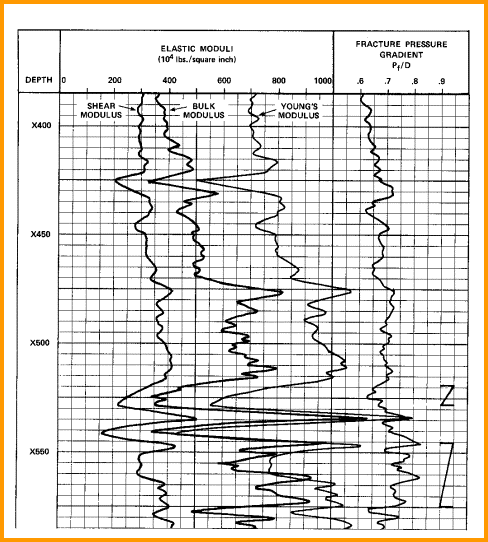Calibrating Fracture Pressure Gradient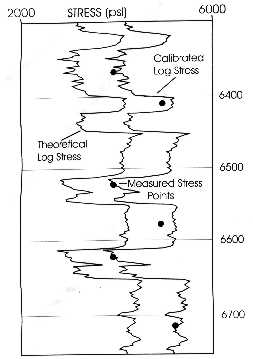Because so many assumptions are made in computing elastic constants and pressure gradients, calibration is essential. If all the corrections for frequency, gas, dynamic to static, anisotropy, and so on are performed first, the correction factors may be relatively small. The cause for error may even become apparent and the correction might be made to Poisson's Ratio or overburden pressure. However, the more usual case is that the cause is unknown.

A common correction method is to compare log analysis stress profiles with individual results from single or multiple mini-fracs. The correction may be a linear shift of the log derived curve, such as the example in the log at the right.

Mini-fracs or leak-off tests should be run to verify that the computed fracture pressure is close to the leak-off pressure. These tests are also called pump-in tests. If they are not equal, then there is anisotropy or tectonic stress (Pext). Alternatively, some of the data or assumptions that went into calculating Po, Pp, Pfrac, PR, or ALPHA might be wrong. The math should be iterated to obtain a good match to the mini-frac without resorting to unreasonable gradients or rock properties.Leak-off pressure test versus time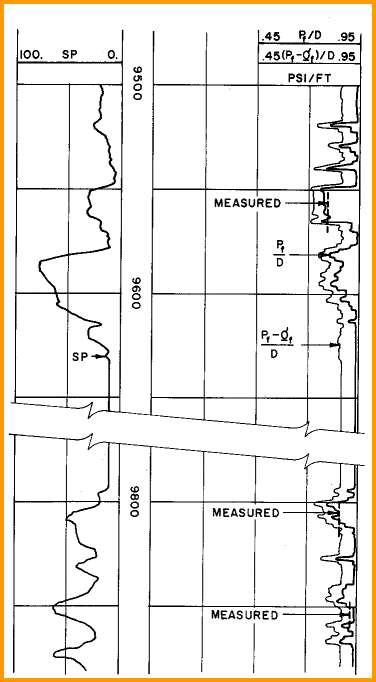Fracture pressure gradient log showing shift to match measured
closure stress (black dots).

An alternative fracture pressure approach ignores all the log derived data. Since the calculation must be calibrated anyway, why not calibrate directly to available mini-fracs, and ignore the log data? Mike Cleary proposed the following equation:
19: Pfrac = A * Po + B * Pp + C

Where: A, B, C are constants derived from regression with pressures from mini-fracs.

This approach only works when sufficient tests exist over a moderate depth range. It is of course useless where there is no test data. It also loses a lot in translation, since the underlying physics is hidden from view.

Because of the major improvements in measuring shear sonic travel time that have occurred in the last 10 years or so, and the recognition and measurement of anisotropy in acoustic properties, many of Cleary's complaints about elastic properties from acoustics have disappeared. His ABC method may not need to be invoked as often as in the past.

My advice is try the log analysis method first if decent modern data is available. Calibrate results to mini-fracs. Try and find the sources of errors and fix them. When there is no decent log data, use Cleary's ABC approach.Calculating Fracture Extent
Programs for fracture design are commonly called "frac height" programs, but fracture extent (width) and fracture aperture are also vital results. The math for this software is a little complicated for this Chapter, and we assume you have a commercial software product to perform the work. Accurate elastic constants and pressure values derived as in previous Sections will be needed, and calibration will still be required.

The modern use of the elastic properties and fracture pressure gradient data in the computer creates some very impressive colour displays to present the hydraulic fracture design to potential customers. The same data can be entered into 3-D modeling programs and compared to real frac jobs to assist in frac job optimization.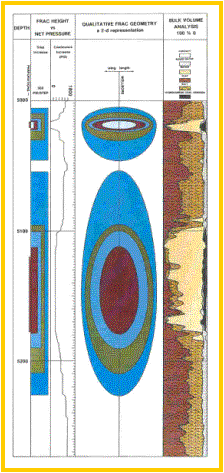FracHite log (left)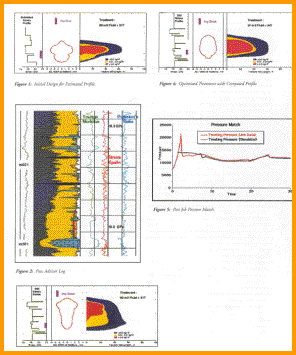Fracture optimization modelGamma Ray Logging to Confirm Fracture Placement
To determine where a hydraulic fracture really goes into a formation, some of the propping material can be coated with radioactive tracer materials. After the fracture stimulation treatment is finished, a standard gamma ray log is run to locate the tracer elements. A base log must be run before the fracture stimulation to make comparison easy.

The fracture height determined from observation of the gamma ray log is used in type-curve-fit or simulation software, with the treatment placement pressure curve, to calculate fracture length (depth of penetration). The fluid plus proppant volume is used in the simulation to calculate fracture width (aperture).

Some fracturing companies use a spectral gamma ray logging tool to locate different radioactive tracer elements that have been applied to different sized propping materials. The finer sized proppants will show the deepest penetration, with coarser material being deposited closer to the wellbore. The spectralog gives a 3-D image of the fracture length, height, and width (aperture). These tracers have very short half-lives (hours or days) so no permanent radioactive signature is created).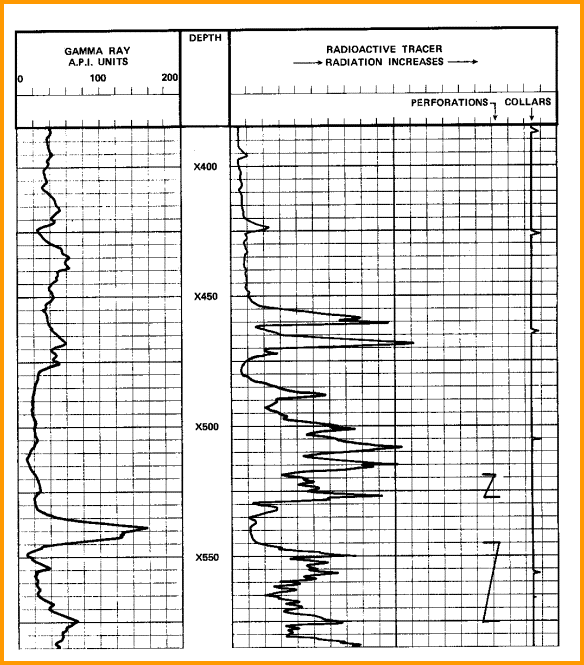Post-frac radioactive tracer log with natural gamma ray in Track 1 recorded before stimulation. Tracer log in Tracks 2 and 3 shows some placement into both sets of pwefs, but alsp above upper perfs, possibly due to channel in cement behind casing.

The gamma ray curve amplitude is a qualitative indicator of fracture width (a

perture) since the quantity of radioactivity is proportional to the volume of proppant that carries the tracer elements.

Note that after a period of production from any reservoir, there may be a permanent radioactive anomaly caused by precipitation of uranium salts. A gamma ray log run in this situation helps to identify where fluid flow is occurring. Some remedial action may be possible if flow is not as expected. Some naturally fractured reservoirs show this anomaly before production. In this case, the precipitation occurred during migration of the hydrocarbon.

If a producing or naturally fractured reservoir is to be hydraulically fractured, a baseline gamma ray log should be run before the job. The post-frac tracer log should be compared to this baseline, rather than the original open hole gamma ray log.Determining Fracture Orientation
As mentioned above, when formation pressure is isotropic (equal in all directions), the tectonic stress is zero and Pfrx equals Pfry. In this situation, the borehole is round and spalling of the formation is either non-existent or equal in all directions. In stressed regions, such as in the Rocky Mountains, the borehole will erode to an oval shape. The minimum diameter shows the direction of maximum stress and the maximum diameter shows the direction of minimum stress..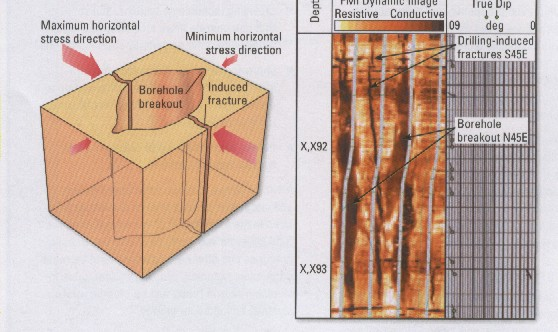Borehole shape indicates stress direction – maximum stress in direction of minimum hole
diameter. Formation microscanner and dipmeters have oriented caliper data.

Many modern logs have an X and Y axis caliper, but not all of them are oriented to true north. When directional data is recorded, as with dipmeters and many modern resistivity tools, the X and Y orientations are known, Statistical plots are helpful in choosing the dominant direction).Borehole diameter indicates stress direction - this example is from India where the minimum
stress direction is NE - SW.A hydraulic fracture will usually penetrate the formation in a plane normal to minimum stress, or parallel to the plane of maximum stress. Any stress anisotropy (tectonic stress) will cause the fracture to be other than vertical.

Natural fractures take the same directions as hydraulic fractures, indicated again by the borehole shape. In addition, the high angle dips seen on an open hole dipmeter or image log, will also indicate this preferential direction. Since most hydraulic fracture jobs are run in casing, it is not possible to run a dipmeter or caliper survey to find the orientation of a hydraulic fracture. The preferential direction can be predicted from previous open hole data. Dipmeter and caliper data can be displayed on rose diagrams to illustrate preferential directions.

If an azimuthal gamma ray log existed, the fracture orientation could be located by a tracer survey. I am not aware that such a tool exists, but it would not be difficult to design one..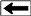Azimuth frequency (rose diagram) plots show direction of dips seen on dipmeter and image logs. When steep dips caused by fractures are isolated from lower angle bedding dips, the direction of maximum stress xan be determined. In this case, the direction is N30E.

Stress direction is not constant over geological time scales. Differences in the direction of induced fractures (present day stress direction), open fractures (some time ago), healed fractures (older than open fractures), and small faults (could be any age) will help to show the stress history of a region. An example log and rose diagrams are shown below.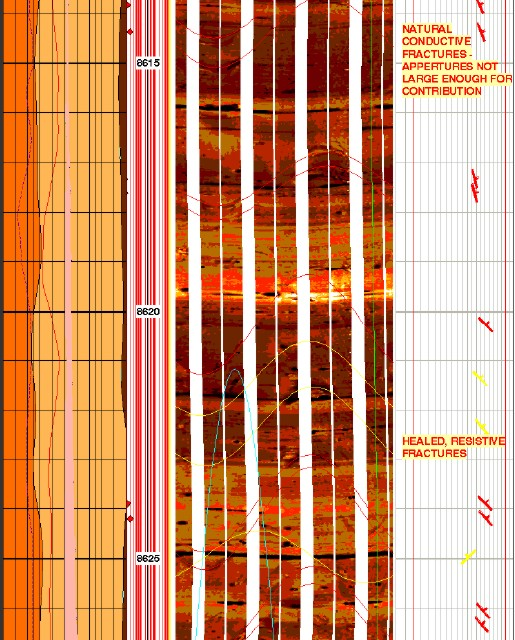Image log in fractured reservoir: gamma ray (left track, shaded red), image track (middle) with open fractures (red sine waves and healed fractures (yellow sine waves), dip track (right) shows red amd yellow dip angle and azimuth. There are no induced fractures in this short interval. Bedding planes are near horizontal. Imagine trying to locate these steep dips without the aid of a computer.

.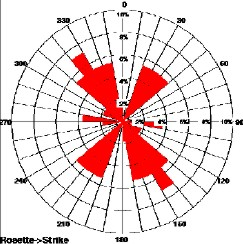Induced fractures (top left) show current stress direction. Open fractures (top right) show stress direction when fractures were created, healed fractures (lower left) show different direction at an earlier phase in geological time, and micro faults (lower right) shows another stress regime was present when the faults occurred.

The newest dipole shear sonic log is also an azimuthal tool with dipole sources set at 90 degrees to each other. The example below shows the shear images for the X and Y directions. This log can be run in open or cased hole.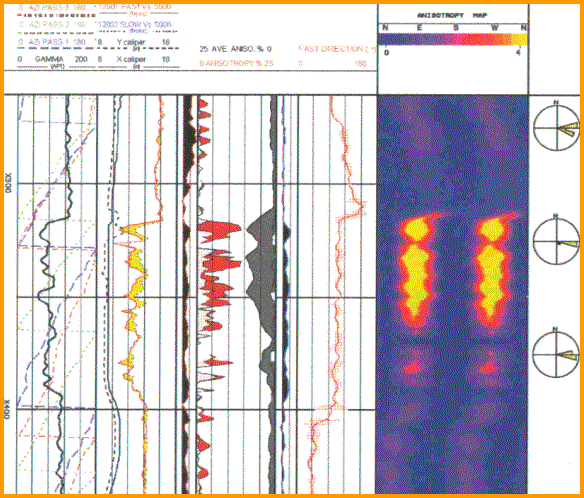Dipole shear image log shows directional stress - the Fast Direction is centered on
90 degrees (east - west) which is also the maximum stress direction.

Resistivity and acoustic image logs also provide assistance in locating fracture orientation before the well is cased.

Page Views ---- Since 01 Jan 2015
Copyright 2023 by Accessible Petrophysics Ltd.
CPH Logo, "CPH", "CPH Gold Member", "CPH Platinum Member", "Crain's Rules", "Meta/Log", "Computer-Ready-Math", "Petro/Fusion Scripts" are Trademarks of the Author